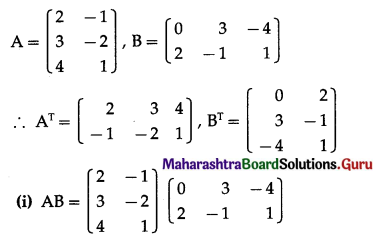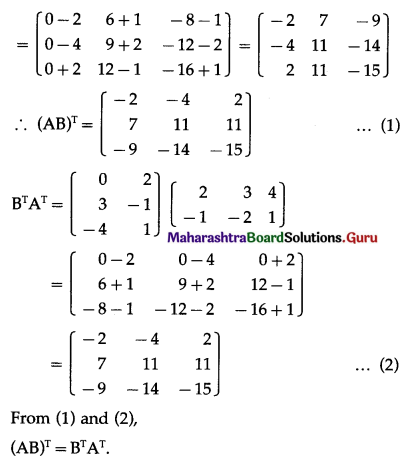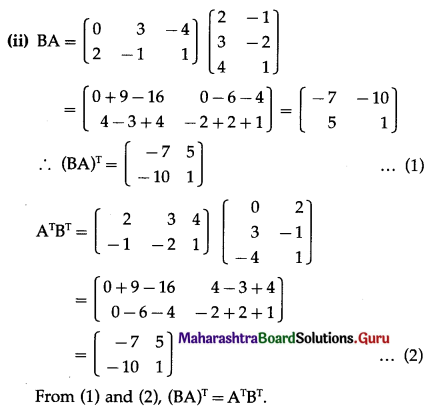Balbharati Maharashtra State Board 12th Commerce Maths Solution Book Pdf Chapter 2 Matrices Ex 2.4 Questions and Answers.

## Maharashtra State Board 12th Commerce Maths Solutions Chapter 2 Matrices Ex 2.4

Question 1.
Find AT, if
(i) A = $$\left[\begin{array}{cc} 1 & 3 \\ -4 & 5 \end{array}\right]$$
(ii) A = $$\left[\begin{array}{ccc} 2 & -6 & 1 \\ -4 & 0 & 5 \end{array}\right]$$
Solution: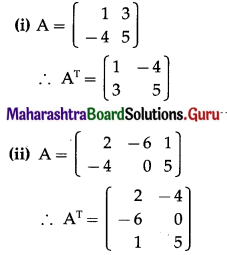Question 2.
If A = [aij]3×3 where aij = 2(i – j). Find A and AT. State whether A and AT both are symmetric or skew-symmetric matrices.
Solution: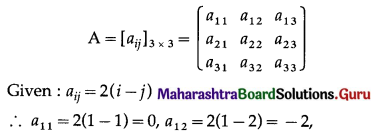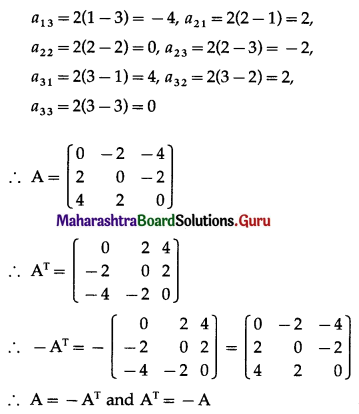Hence, A and AT are both skew-symmetric matrices.Question 3.
If A = $$\left[\begin{array}{cc} 5 & -3 \\ 4 & -3 \\ -2 & 1 \end{array}\right]$$, prove that (AT)T = A.
Solution: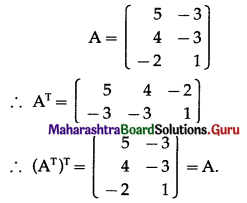Question 4.
If A = $$\left[\begin{array}{ccc} 1 & 2 & -5 \\ 2 & -3 & 4 \\ -5 & 4 & 9 \end{array}\right]$$, prove that AT = A.
Solution: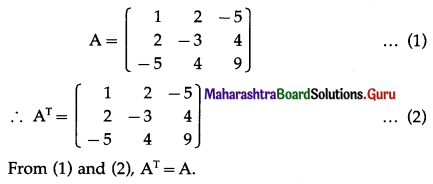Question 5.
If A = $$\left[\begin{array}{cc} 2 & -3 \\ 5 & -4 \\ -6 & 1 \end{array}\right]$$, B = $$\left[\begin{array}{cc} 2 & 1 \\ 4 & -1 \\ -3 & 3 \end{array}\right]$$, C = $$\left[\begin{array}{cc} 1 & 2 \\ -1 & 4 \\ -2 & 3 \end{array}\right]$$, then show that
(i) (A + B)T = AT + BT
(ii) (A – C)T = AT – CT
Solution: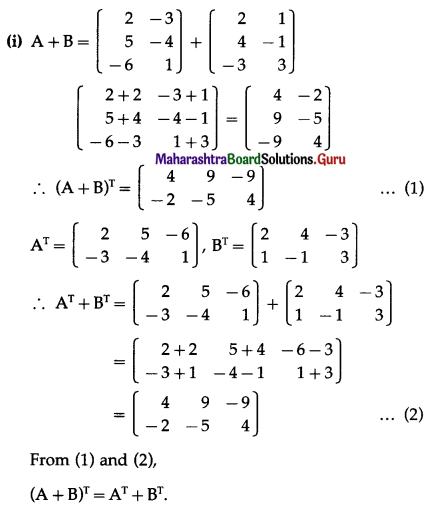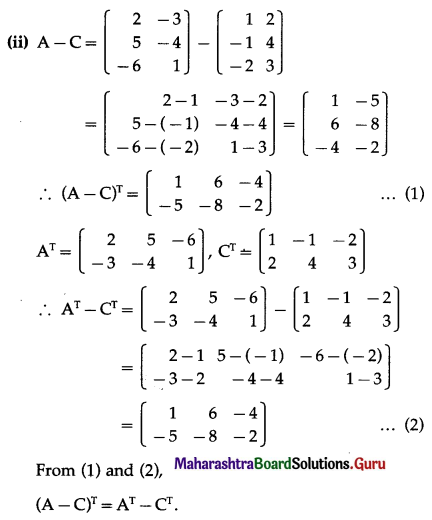Question 6.
If A = $$\left[\begin{array}{cc} 5 & 4 \\ -2 & 3 \end{array}\right]$$ and B = $$\left[\begin{array}{cc} -1 & 3 \\ 4 & -1 \end{array}\right]$$, then find CT, such that 3A – 2B + C = I, where I is the unit matrix of order 2.
Solution: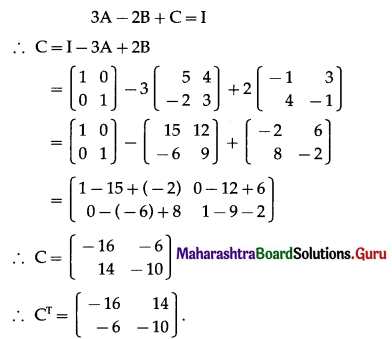Question 7.
If A = $$\left[\begin{array}{ccc} 7 & 3 & 0 \\ 0 & 4 & -2 \end{array}\right]$$, B = $$\left[\begin{array}{ccc} 0 & -2 & 3 \\ 2 & 1 & -4 \end{array}\right]$$, then find
(i) AT + 4BT
(ii) 5AT – 5BT
Solution: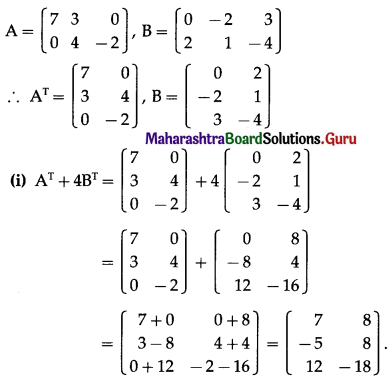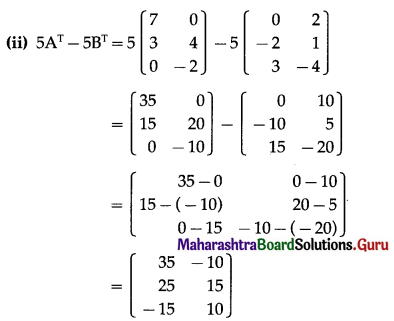Question 8.
If A = $$\left[\begin{array}{lll} 1 & 0 & 1 \\ 3 & 1 & 2 \end{array}\right]$$, B = $$\left[\begin{array}{lll} 2 & 1 & -4 \\ 3 & 5 & -2 \end{array}\right]$$ and C = $$\left[\begin{array}{ccc} 0 & 2 & 3 \\ -1 & -1 & 0 \end{array}\right]$$, verify that (A + 2B + 3C)T = AT + 2BT + 3CT
Solution: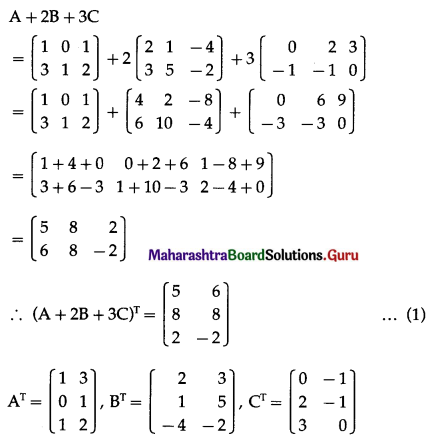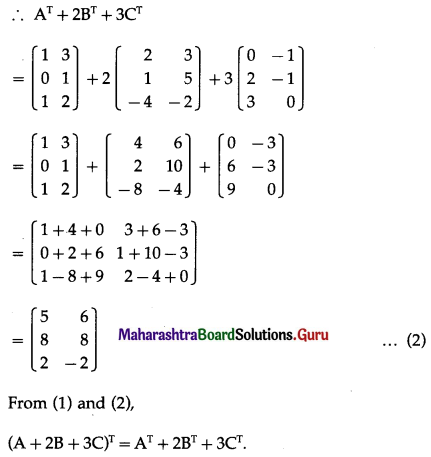Question 9.
If A = $$\left[\begin{array}{ccc} -1 & 2 & 1 \\ -3 & 2 & -3 \end{array}\right]$$ and B = $$\left[\begin{array}{cc} 2 & 1 \\ -3 & 2 \\ -1 & 3 \end{array}\right]$$, prove that (A + BT)T = AT + B.
Solution: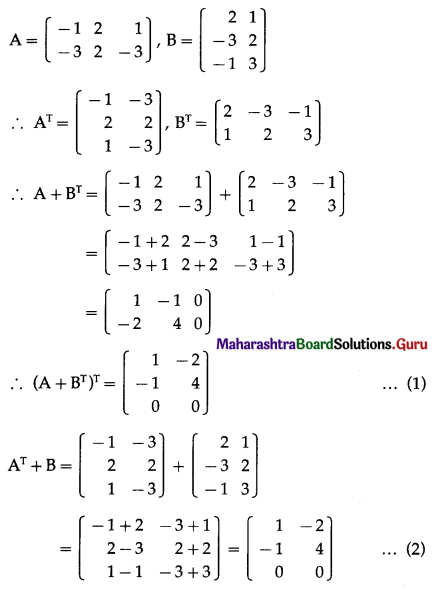From (1) and (2),
(A + BT)T = AT + B.

Question 10.
Prove that A + AT is symmetric and A – AT is a skew-symmetric matrix, where
(i) A = $$\left[\begin{array}{ccc} 1 & 2 & 4 \\ 3 & 2 & 1 \\ -2 & -3 & 2 \end{array}\right]$$
(ii) A = $$\left[\begin{array}{ccc} 5 & 2 & -4 \\ 3 & -7 & 2 \\ 4 & -5 & -3 \end{array}\right]$$
Solution: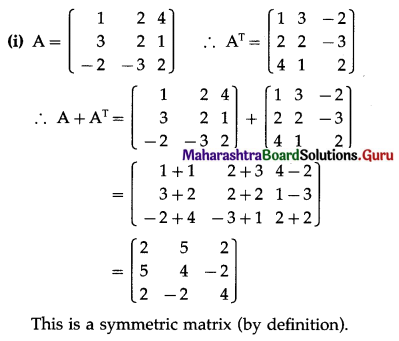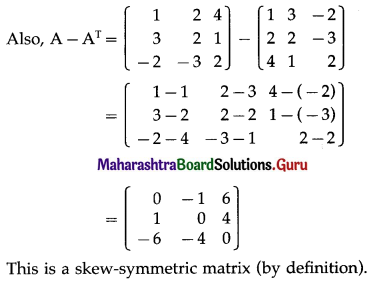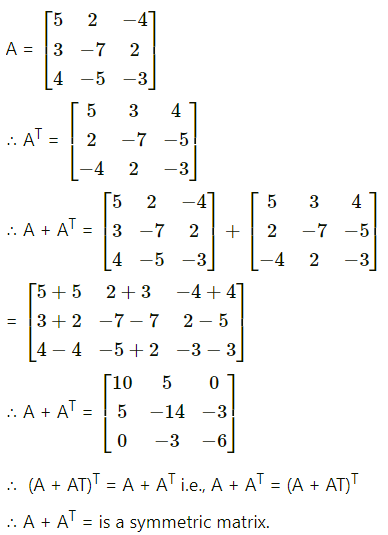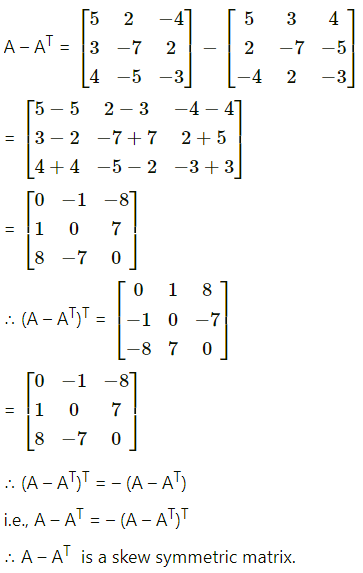Question 11.
Express each of the following matrix as the sum of a symmetric and a skew-symmetric matrix:
(i) $$\left[\begin{array}{ll} 4 & -2 \\ 3 & -5 \end{array}\right]$$
(ii) $$\left[\begin{array}{ccc} 3 & 3 & -1 \\ -2 & -2 & 1 \\ -4 & -5 & 2 \end{array}\right]$$
Solution: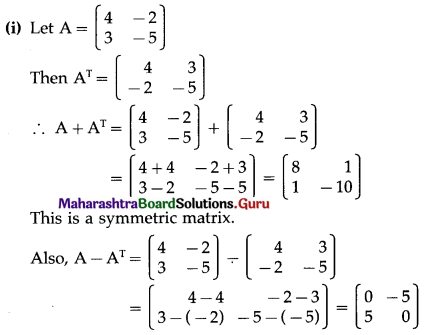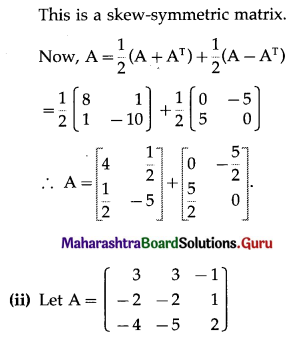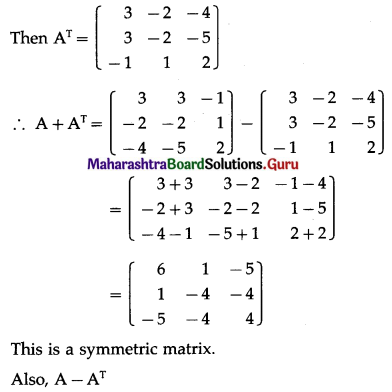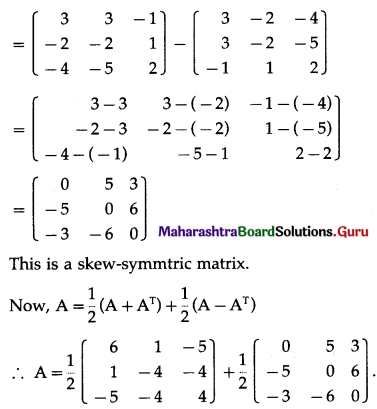Question 12.
If A = $$\left[\begin{array}{cc} 2 & -1 \\ 3 & -2 \\ 4 & 1 \end{array}\right]$$ and B = $$\left[\begin{array}{ccc} 0 & 3 & -4 \\ 2 & -1 & 1 \end{array}\right]$$, verify that
(i) (AB)T = BTAT
(ii) (BA)T = ATBT
Solution: